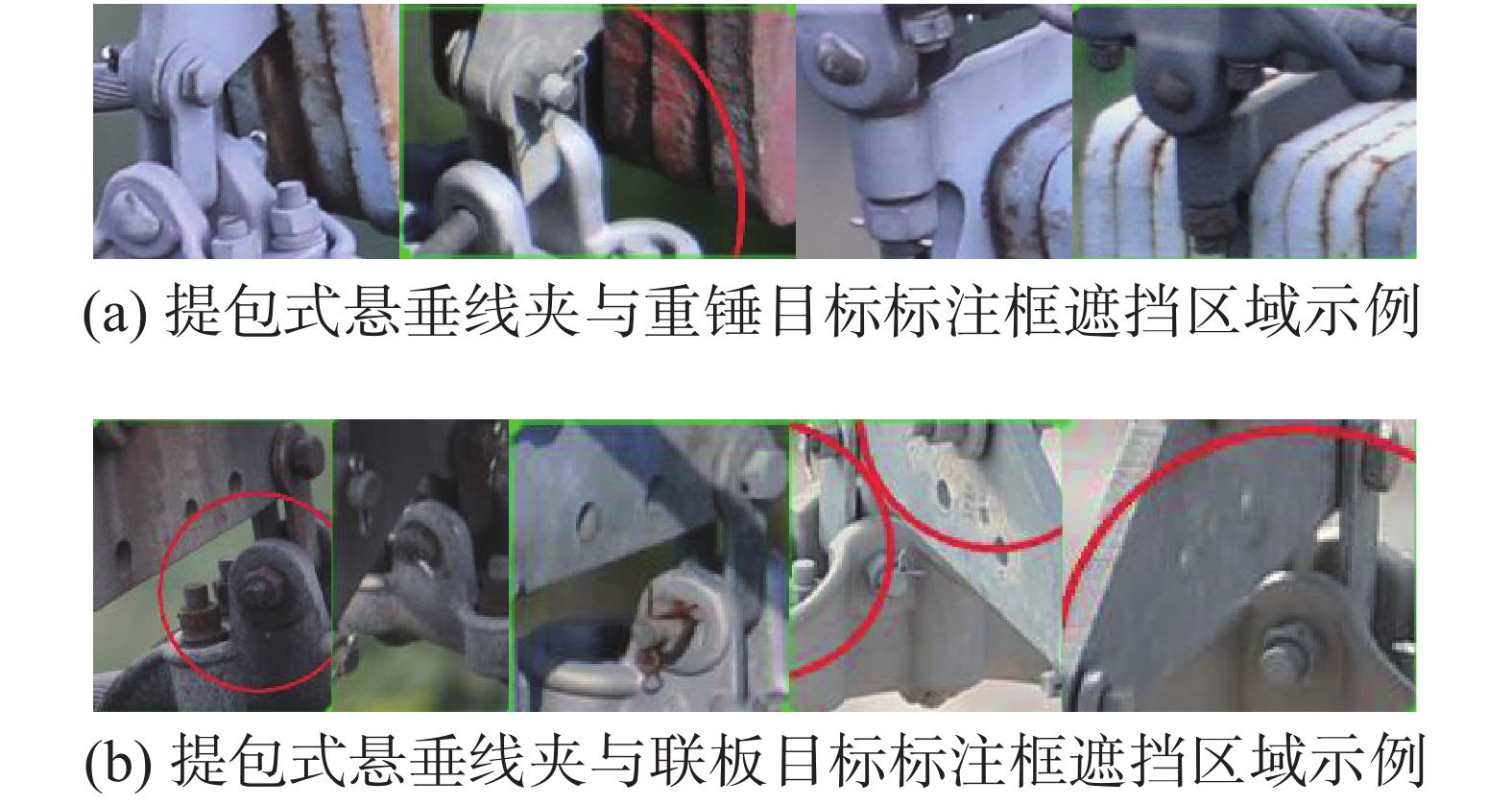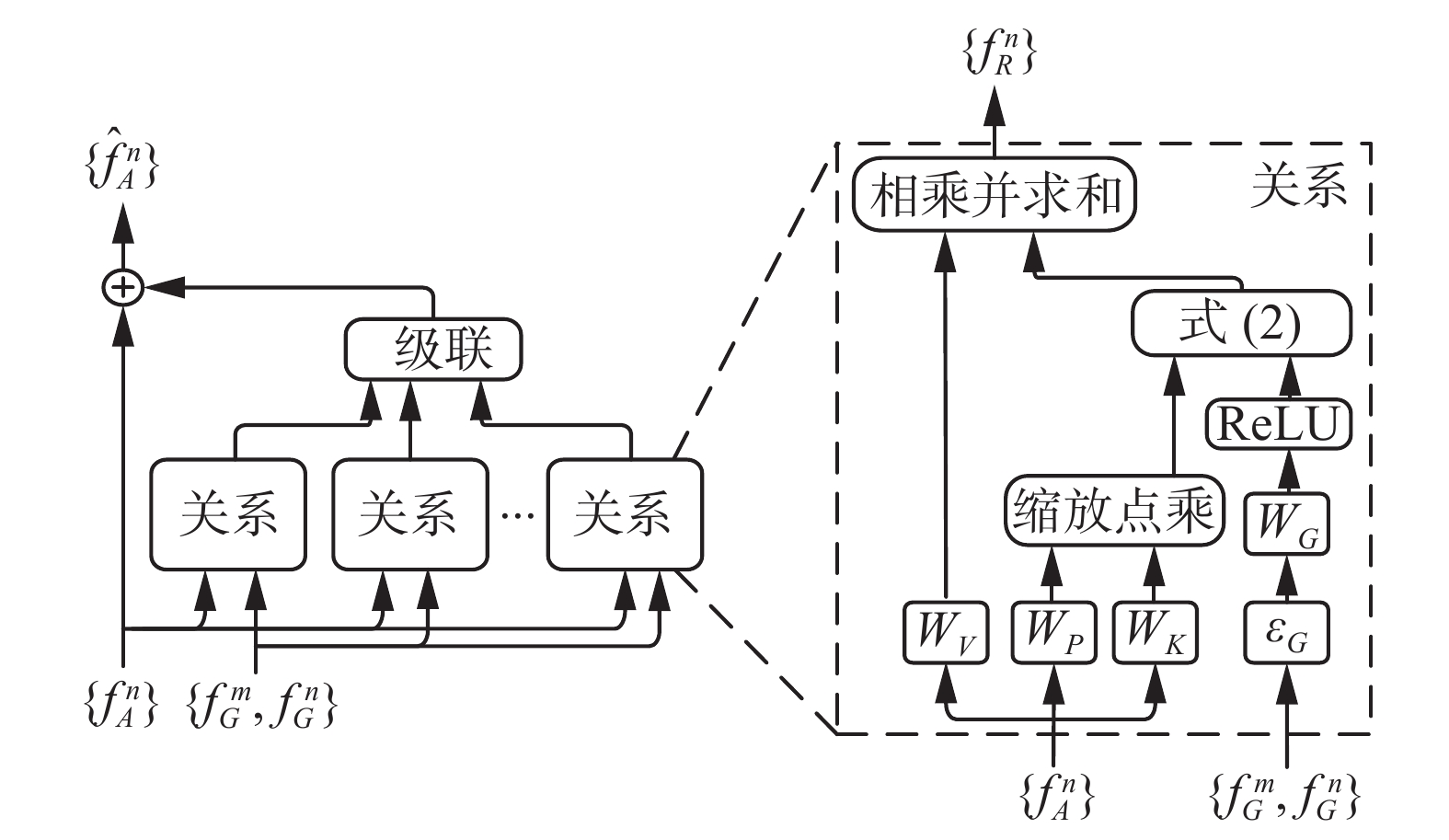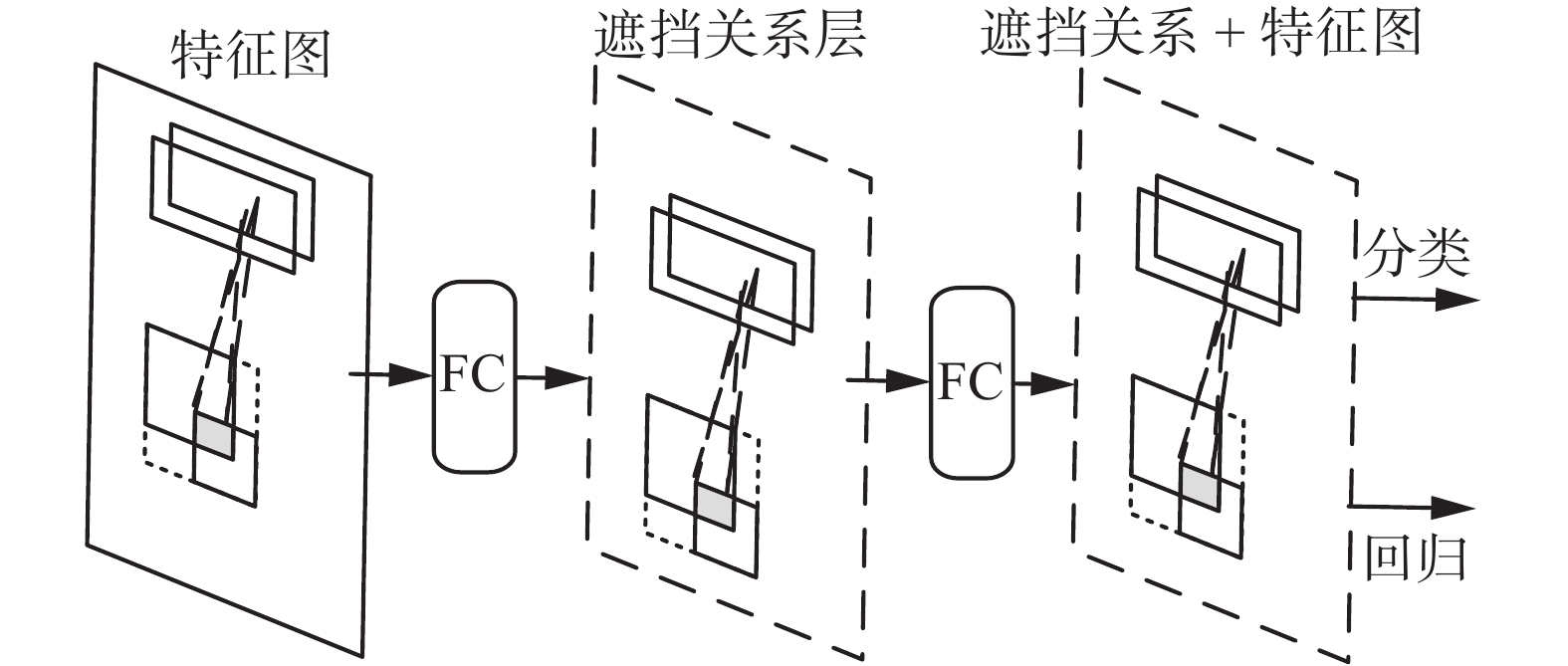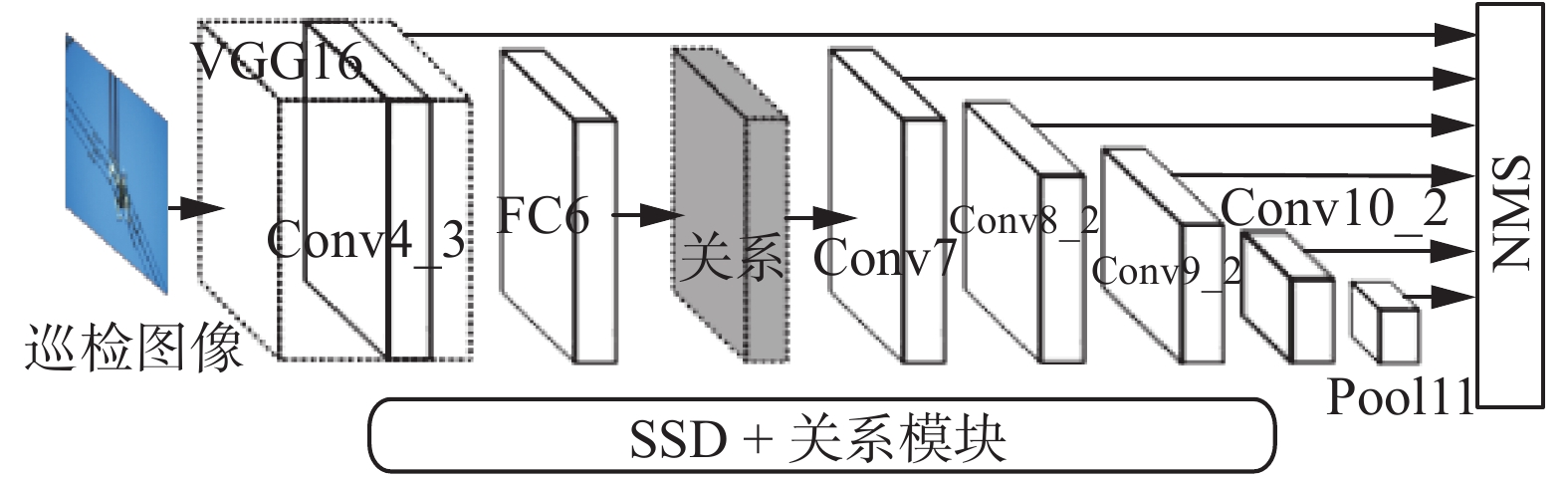﻿ 嵌入遮挡关系模块的SSD模型的输电线路图像金具检测
«上一篇文章快速检索 高级检索

 智能系统学报2020, Vol. 15Issue (4): 656-662  DOI: 10.11992/tis.2020010080

### 引用本文ZHAO Zhenbing, JIANG Aixue, QI Yincheng, et al. Fittings detection in transmission line images with SSD model embedded occlusion relation module[J]. CAAI Transactions on Intelligent Systems, 2020, 15(4): 656-662. DOI: 10.11992/tis.202001008.### 文章历史

1. 华北电力大学 电气与电子工程学院，河北 保定 071003;
2. 华北电力大学 控制与计算机工程学院，河北 保定 071003

Fittings detection in transmission line images with SSD model embedded occlusion relation module
ZHAO Zhenbing 1, JIANG Aixue 1, QI Yincheng 1, ZHANG Wei 1, ZHAO Wenqing 2
1. School of Electrical and Electronic Engineering, North China Electric Power University, Baoding 071003, China;
2. School of Control and Computer Engineering, North China Electric Power University, Baoding 071003, China
Abstract: In order to improve the accuracy of the deep learning object detection model in the automatic detection of transmission fittings, aiming at the problem of inaccurate detection and location of fittings due to the inevitable extensive intersection between the groundtruth boxes of fittings in the fittings dataset, this article proposes a description method of the occlusion relation between the objects, so as to regularly describe the mutual occlusion between the objects by using the similarity of the intersection area as the context information of the fittings. The occlusion relation module is designed and embedded in the single shot multibox detector (SSD) model. In order to verify the effectiveness of the SSD model embedded with the occlusion relation module, eight kinds of small objects with intersecting groundtruth boxes are selected for experiments, and the object number of the training set and the test set of the fittings dataset used in the experiment is 6271 and 1713 respectively. The experiments show that the mean average precision (mAP) of the original SSD model is 72.10%, the mAP of the SSD model embedded in the occlusion relation module is 76.56%, and the performance is improved by 4.46%.
Key words: transmission line fittings    occlusion    occlusion relationship description    occlusion relationship module    single shot multibox detector    groundtruth box    object detection    deep learning

1 研究方法Download: 图 1 金具检测数据集中目标标注框遮挡区域示例 Fig. 1 Example of occluded area of object annotation box in fittings detection dataset

1.1 遮挡关系描述

 ${\left[\dfrac{{2{x_{mn}}}}{{{x_m} + {x_n}}}\; \dfrac{{2{y_{mn}}}}{{{y_m} + {y_n}}}\; \dfrac{{{w_{mn}}}}{{{w_m}}}\; \dfrac{{{h_{mn}}}}{{{h_m}}}\right]^{\rm{T}}}$ (1)

1)输入为目标mn坐标 $({x_m},{y_m},{w_m},{h_m})$ $({x_n},{y_n}, {w_n},{h_n})$ ，计算目标mn的中心点坐标 $({x_{mo}},{y_{mo}})$ $({x_{no}},{y_{no}})$

2)判断目标mn存在相互遮挡的条件为

 $\begin{array}{l} \left| {{x_{mo}} - {x_{no}}} \right| \leqslant {\dfrac{1}{2}}({w_m} + {w_n})\\ \left| {{y_{mo}} - {y_{no}}} \right| \leqslant {\dfrac{1}{2}}({h_m} + {h_n}) \end{array}$ (2)

3)输出相交区域坐标 $({x_{mn}},{y_{mn}},{w_{mn}},{h_{mn}})$ 。当目标mn未遮挡时，相交区域坐标为(0,0,0,0)；当两目标存在相互遮挡时，通过式（3）计算相交区域坐标：

 $\begin{array}{c} {x_{mn}} = \max ({x_m},{x_n})\\ {y_{mn}} = \max ({y_m},{y_n})\\ {w_{mn}} = \min ({w_m} + {x_m},{w_n} + {x_n}) - {x_{mn}}\\ {h_{mn}} = \min ({h_m} + {y_m},{h_n} + {y_n}) + {y_{mn}} \end{array}$ (3)
1.2 遮挡关系模块设计

 ${f_R}(n) = \displaystyle \sum\limits_m {{\omega ^{mn}} \cdot ({W_V} \cdot f_A^m)}$ (4)

 ${\omega ^{mn}} = \frac{{\omega _G^{mn} \cdot \exp (\omega _A^{mn})}} {{ \displaystyle \sum\nolimits_k {\omega _G^{kn} \cdot \exp (\omega _A^{kn})} }}$ (5)

 $\omega _A^{mn} = \dfrac{{{\rm{dot}}({W_K}f_A^m,{W_P}f_A^n)}}{\sqrt {{d_k}} }$ (6)

 $\omega _G^{mn} = \max \{ 0,{W_G} \cdot \varepsilon _G}(f_G^m,f_G^n)\}$ (7)

 ${\left[\lg \Bigg(\dfrac{{\left|{x_m} - {x_n}\right|}}{{{w_m}}}\Bigg)\;\;\; \lg \Bigg(\dfrac{{\left|{y_m} - {y_n}\right|}}{{{h_m}}}\Bigg)\;\;\; \lg \Bigg(\dfrac{{{w_n}}}{{{w_m}}}\Bigg)\;\;\; \lg \Bigg(\dfrac{{{h_n}}}{{{h_m}}}\Bigg)\right]^{\rm{T}}}$ (8)

 $\hat f_A^n = f_A^n + {\rm{Contact}}[f_R^1(n),f_R^{\rm{2}}(n),\cdots,f_R^N(n)]$ (9)Download: 图 2 遮挡关系模块结构图 Fig. 2 Occlusion relationship module structural diagram
1.3 嵌入遮挡关系模块的SSD模型Download: 图 3 遮挡关系模块嵌入两层全连接层中间的示意图 Fig. 3 Schematic diagram of occlusion relationship module embedded in the middle of two full connection layers

SSD模型以VGG16网络为基础模型，并且在基础模型后新增了卷积层以获得丰富的特征图用于目标检测，最后基于模型的Conv4_3、Conv6(FC6)、Conv7(FC7)、Conv8_2、Conv9_2、Conv10_2、Conv11_2这7个层提取的特征图分别做分类和回归，其中Conv6(FC6)和Conv7(FC7)两个卷积层大小维度相同。基于此，将遮挡关系模块灵活地嵌入这两个层之间，完成遮挡关系模块的嵌入，最终SSD模型可以在融合遮挡关系模块的Conv7层进行分类和回归，其示意如图4Download: 图 4 嵌入遮挡关系模块的SSD模型结构 Fig. 4 SSD model structure embedded in occlusion relationship module
2 实验结果及分析表 1 遮挡关系模块加入前后金具的AP结果 Tab.1 Results of AP before and after adding occlusion relationship module表 2 检测方法性能对比 Tab.2 Performance comparison results of detection methodsDownload: 图 5 嵌入遮挡关系模块前后SSD模型检测结果 Fig. 5 Detection results of SSD model before and after embedding occlusion relationship module
3 结束语

  赵强, 左石. 输电线路金具理论与应用[M]. 北京: 中国电力出版社, 2013: 2−12. (0)  傅博, 姜勇, 王洪光, 等. 输电线路巡检图像智能诊断系统[J]. 智能系统学报, 2016, 11(1): 70-77. FU Bo, JIANG Yong, WANG Hongguang, et al. Intelligent diagnosis system for patrol check images of power transmission lines[J]. CAAI transactions on intelligent systems, 2016, 11(1): 70-77. DOI:10.11992/tis.201503043 (0)  王身丽, 黄力, 侯金华, 等. 基于最大熵的复合绝缘子表面水珠图像分割算法研究[J]. 通信电源技术, 2018, 35(1): 48-50. WANG Shenli, HUANG Li, HOU Jinhua, et al. Study on segmentation method of water drops image on composite insulator based on maximum entropy[J]. Telecom power technology, 2018, 35(1): 48-50. (0)  金立军, 胡娟, 闫书佳. 基于图像的高压输电线间隔棒故障诊断方法[J]. 高电压技术, 2013, 39(5): 1040-1045. JIN Lijun, HU Juan, YAN Shujia. Method of spacer fault diagnose on transmission line based on image procession[J]. High voltage engineering, 2013, 39(5): 1040-1045. DOI:10.3969/j.issn.1003-6520.2013.05.003 (0)  陈晓娟, 吴英石, 赵亮. 基于随机Hough变换的OPGW防震锤识别[J]. 黑龙江电力, 2010, 32(1): 1-2. CHEN Xiaojuan, WU Yingshi, ZHAO Liang. Identification of OPGW vibration damper based on random Hough transformation[J]. Heilongjiang electric power, 2010, 32(1): 1-2. DOI:10.3969/j.issn.1002-1663.2010.01.001 (0)  江慎旺, 许廷发, 张增, 等. 基于无人机的输电网故障跳线联板识别[J]. 液晶与显示, 2016, 31(12): 1149-1155. JIANG Shenwang, XU Tingfa, ZHANG Zeng. Recognition algorithm for fault jumper connection plate of transmission network based on UAV[J]. Chinese journal of liquid crystals and displays, 2016, 31(12): 1149-1155. DOI:10.3788/YJYXS20163112.1149 (0)  翟永杰, 王迪, 赵振兵. 基于目标建议与结构搜索的绝缘子识别方法[J]. 华北电力大学学报, 2016, 43(4): 66-71. ZHAI Yongjie, WANG Di, ZHAO Zhenbing. Recognition method of insulator based on object proposals and structure research[J]. Journal of North China Electric Power University, 2016, 43(4): 66-71. (0)  王森. 输电线路图像上防震锤检测算法研究[D]. 北京: 北京交通大学, 2017: 21−44. WANG Sen. Research on algorithms of vibration damper detection on power line image[D]. Beijing: Beijing Jiaotong University, 2017: 21−44. (0)  刘永姣. 输电线路绝缘子视觉跟踪技术应用研究[D]. 成都: 电子科技大学, 2017: 35−45. LIU Yongjiao. Research on application of visual tracking technology of insulator for transmission line[D]. Chengdu: University of Electronic Science and Technology of China, 2017: 35−45. (0)  刘召, 张黎明, 耿美晓, 等. 基于改进的Faster R-CNN高压线缆目标检测方法[J]. 智能系统学报, 2019, 14(4): 627-634. LIU Zhao, ZHANG Liming, GENG Meixiao, et al. Object detection of high-voltage cable based on improved Faster R-CNN[J]. CAAI transactions on intelligent systems, 2019, 14(4): 627-634. DOI:10.11992/tis.201905026 (0)  汤踊, 韩军, 魏文力, 等. 深度学习在输电线路中部件识别与缺陷检测的研究[J]. 电子测量技术, 2018, 41(6): 60-65. TANG Yong, HAN Jun, WEI Wenli, et al. Research on part recognition and defect detection of transmission line in deep learning[J]. Electronic measurement technology, 2018, 41(6): 60-65. (0)  高强, 廉启旺. 航拍图像中绝缘子目标检测的研究[J]. 电测与仪表, 2019, 56(5): 119-123. GAO Qiang, LIAN Qiwang. Research on target detection of insulator in aerial image[J]. Electrical measurement and instrumentation, 2019, 56(5): 119-123. (0)  赵振兵, 崔雅萍, 戚银城, 等. 基于改进的 R-FCN 航拍巡线图像中的绝缘子检测方法[J]. 计算机科学, 2019, 46(3): 159-163. ZHAO Zhenbing, CUI Yaping, QI Yincheng, et al. Detection method of insulator in aerial inspection image based on modified R-FCN[J]. Computer science, 2019, 46(3): 159-163. DOI:10.11896/j.issn.1002-137X.2019.03.024 (0)  赵文清, 程幸福, 赵振兵, 等. 注意力机制和Faster RCNN相结合的绝缘子识别[J]. 智能系统学报, 2020, 15(1): 92-98. ZHAO Wenqing, CHENG Xingfu, ZHAO Zhenbing, et al. Insulator recognition based on attention mechanism and Faster RCNN[J]. CAAI transactions on intelligent systems, 2020, 15(1): 92-98. DOI:10.11992/tis.201907023 (0)  张东, 邱翔, 曹成功, 等. 结合聚合通道和复频域特征的防震锤检测算法[J]. 计算机技术与发展, 2020(2): 1-7. ZHANG Dong, QIU Xiang, CAO Chenggong, et al. Algorithm of vibration damper detection combined with aggregation channel and complex frequency domain features[J]. Computer technology and development, 2020(2): 1-7. DOI:10.3969/j.issn.1673-629X.2020.02.001 (0)  LIU W, ANGUELOV D, ERHAN D, et al. SSD: single shot multibox detector[C]//European Conference on ComputerVision. Amsterdam, Netherlands, 2016: 21−37. (0)  赵文清, 周震东, 翟永杰. 基于反卷积和特征融合的SSD小目标检测算法[J]. 智能系统学报, 2020, 15(2): 310-316. ZHAO Wenqing, ZHOU Zhendong, ZHAI Yongjie. SSD small target detection algorithm based on deconvolution and feature fusion[J]. CAAI transactions on intelligent systems, 2020, 15(2): 310-316. DOI:10.11992/tis.201905035 (0)  伍鹏瑛, 张建明, 彭建, 等. 多层卷积特征的真实场景下行人检测研究[J]. 智能系统学报, 2019, 14(2): 306-315. WU Pengying, ZHANG Jianming, PENG Jian, et al. Research on pedestrian detection based on multi-layer convolution feature in real scene[J]. CAAI transactions on intelligent systems, 2019, 14(2): 306-315. DOI:10.11992/tis.201710019 (0)  CHEN X, GUPTA A. Spatial memory for context reasoning in object detection[C]//Proceedings of the IEEE International Conference on Computer Vision, Venice, Italy, 2017: 4086−4096. (0)  MOTTAGHI R, CHEN X, LIU X, et al. The role of context for object detection and semantic segmentation in the wild[C]//Proceedings of the IEEE Conference on Computer Vision and Pattern Recognition, Columbus, USA, 2014: 891−898. (0)  LIU Y, WANG R, SHAN S, et al. Structure inference net: object detection using scene-level context and instance-level relationships[C]//Proceedings of the IEEE Conference on Computer Vision and Pattern Recognition, Salt Lake City, USA, 2018: 6985−6994. (0)  HU H, GU J, ZhANG Z, et al. Relation networks for object detection[C]//Proceedings of the IEEE Conference on Computer Vision and Pattern Recognition, Salt Lake City, USA, 2018: 3588−3597. (0)  VASWANI A, SHAZEER N, PARMAR N, et al. Attention is all you need[C]//Advances in Neural Information Processing Systems. Long Beach, USA, 2017: 5998−6008. (0)  葛园园, 许有疆, 赵帅, 等. 自动驾驶场景下小且密集的交通标志检测[J]. 智能系统学报, 2018, 13(3): 366-372. GE Yuanyuan, XU Youjiang, ZHAO Shuai, et al. Detection of small and dense traffic signs in self-driving scenarios[J]. CAAI transactions on intelligent systems, 2018, 13(3): 366-372. DOI:10.11992/tis.201706040 (0)  FU C Y, LIU W, RANGA A, et al. DSSD: deconvolutional single shot detector[C]//Proceedings of the IEEE Conference on Computer Vision and Pattern Recognition. Hawaii Convention Center, USA, 2017: 2881−2890. (0)